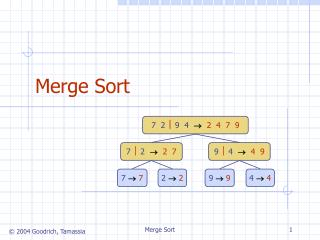DownloadDownload PresentationMerge Sort

# Merge Sort

Télécharger la présentation## Merge Sort

- - - - - - - - - - - - - - - - - - - - - - - - - - - E N D - - - - - - - - - - - - - - - - - - - - - - - - - - -
##### Presentation Transcript

1. 7 2  9 4 2 4 7 9 7  2 2 7 9  4 4 9 7 7 2 2 9 9 4 4 Merge Sort Merge Sort

2. Divide-and conquer is a general algorithm design paradigm: Divide: divide the input data S in two disjoint subsets S1and S2 Recur: solve the subproblems associated with S1and S2 Conquer: combine the solutions for S1and S2 into a solution for S The base case for the recursion are subproblems of size 0 or 1 (sometimes 1 or 2) Merge-sort is a sorting algorithm based on the divide-and-conquer paradigm Like heap-sort It uses a comparator It has O(n log n) running time Unlike heap-sort It does not use an auxiliary priority queue It accesses data in a sequential manner (suitable to sort data on a disk) Divide-and-Conquer (§ 10.1.1) Merge Sort

3. Merge-sort on an input sequence S with n elements consists of three steps: Divide: partition S into two sequences S1and S2 of about n/2 elements each Recur: recursively sort S1and S2 Conquer: merge S1and S2 into a unique sorted sequence Merge-Sort (§ 10.1) AlgorithmmergeSort(S, C) Inputsequence S with n elements, comparator C Outputsequence S sorted • according to C ifS.size() > 1 (S1, S2)partition(S, n/2) mergeSort(S1, C) mergeSort(S2, C) Smerge(S1, S2) Merge Sort

4. Merging Two Sorted Sequences Algorithmmerge(A, B) Inputsequences A and B withn/2 elements each Outputsorted sequence of A  B S empty sequence whileA.isEmpty() B.isEmpty() ifA.first().element()<B.first().element() S.insertLast(A.remove(A.first())) else S.insertLast(B.remove(B.first())) whileA.isEmpty() S.insertLast(A.remove(A.first())) whileB.isEmpty() S.insertLast(B.remove(B.first())) return S • The conquer step of merge-sort consists of merging two sorted sequences A and B into a sorted sequence S containing the union of the elements of A and B • Merging two sorted sequences, each with n/2 elements and implemented by means of a doubly linked list, takes O(n) time Merge Sort

5. Merge-Sort Tree • An execution of merge-sort is depicted by a binary tree • each node represents a recursive call of merge-sort and stores • unsorted sequence before the execution and its partition • sorted sequence at the end of the execution • the root is the initial call • the leaves are calls on subsequences of size 1 or 2 7 2  9 4 2 4 7 9 7  2 2 7 9  4 4 9 Merge Sort

6. Execution Example (cont.) • Recursive call, …, merge, merge 7 2 9 4  3 8 6 11 2 3 4 6 7 8 9 7 2  9 42 4 7 9 3 8 6 1  1 3 6 8 7  22 7 9 4  4 9 3 8 3 8 6 1  1 6 Merge Sort

7. Execution Example (cont.) • Merge 7 2 9 4  3 8 6 11 2 3 4 6 7 8 9 7 2  9 42 4 7 9 3 8 6 1  1 3 6 8 7  22 7 9 4  4 9 3 8 3 8 6 1  1 6 Merge Sort

8. Analysis of Merge-Sort • The height h of the merge-sort tree is O(log n) • at each recursive call we divide in half the sequence, • The overall amount or work done at the nodes of depth i is O(n) • we partition and merge 2i sequences of size n/2i • we make 2i+1 recursive calls • Thus, the total running time of merge-sort is O(n log n) Merge Sort

9. Nonrecursive Merge-Sort public static void mergeSort(Object[] orig, Comparator c) { // nonrecursive Object[] in = new Object[orig.length]; // make a new temporary array System.arraycopy(orig,0,in,0,in.length); // copy the input Object[] out = new Object[in.length]; // output array Object[] temp; // temp array reference used for swapping int n = in.length; for (int i=1; i < n; i*=2) { // each iteration sorts all length-2*i runs for (int j=0; j < n; j+=2*i) // each iteration merges two length-i pairs merge(in,out,c,j,i); // merge from in to out two length-i runs at j temp = in; in = out; out = temp; // swap arrays for next iteration } // the "in" array contains the sorted array, so re-copy it System.arraycopy(in,0,orig,0,in.length); } protected static void merge(Object[] in, Object[] out, Comparator c, int start, int inc) { // merge in[start..start+inc-1] and in[start+inc..start+2*inc-1] int x = start; // index into run #1 int end1 = Math.min(start+inc, in.length); // boundary for run #1 int end2 = Math.min(start+2*inc, in.length); // boundary for run #2 int y = start+inc; // index into run #2 (could be beyond array boundary) int z = start; // index into the out array while ((x < end1) && (y < end2)) if (c.compare(in[x],in[y]) <= 0) out[z++] = in[x++]; else out[z++] = in[y++]; if (x < end1) // first run didn't finish System.arraycopy(in, x, out, z, end1 - x); else if (y < end2) // second run didn't finish System.arraycopy(in, y, out, z, end2 - y); } merge runs of length 2, then 4, then 8, and so on merge two runs in the in array to the out array Merge Sort